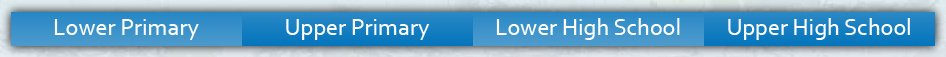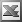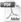Upper High School Enter keywords to search by topic or worksheet description

Worksheets in this category assist provide students with an understanding of analytical geometry through exercises involving linear and quadratic functions,

Worksheet Name Files
Finding Gradient Given Two PointsEquation to a Line: Given Slope & One PointEquation to a Line: Given Two PointsEquations of Perpendicular LinesEquations of Parallel LinesMidpoint of a Line SegmentDistance Between Two PointsQuadratic Equations: Plotting Points & GraphingQuadratic Equations: Curve SketchingCritical PointsTangents & Normals to CubicsTangents & Normals to QuadraticsTangents & Normals to Trig FunctionsTransformation of Periodic Functions: DEMONSTRATION